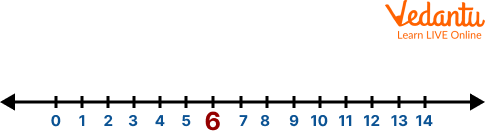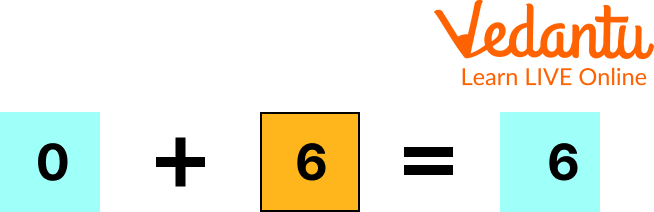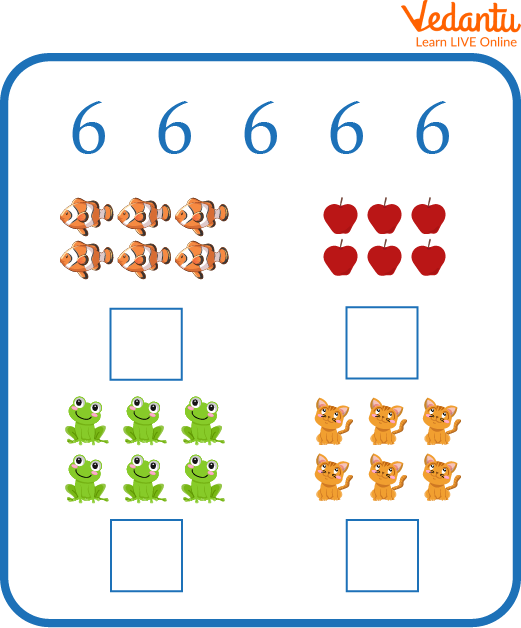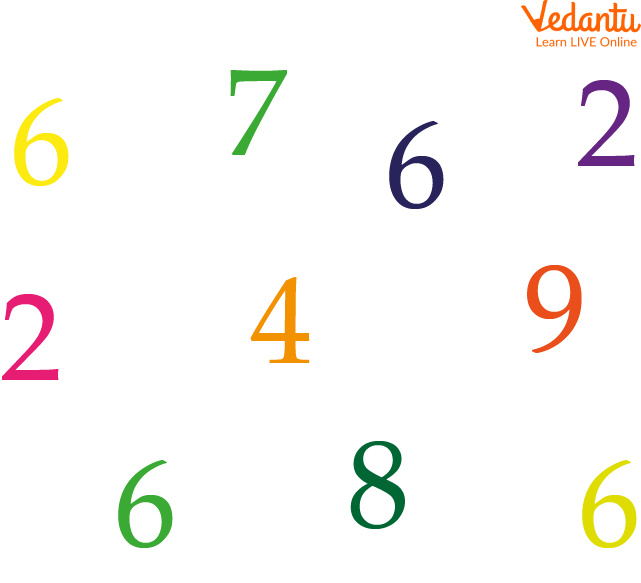Courses
Courses for Kids
Free study material
Free LIVE classes
More

# Learn Number 6## Overview of the Number 6

Are you stuck thinking about how to make your child aware of the need to learn to write the number 6? If so, then you are at the perfect spot. This article will assist you in teaching number 6 to your child. Here, we will discuss the number 6, its different writing forms, its representation on the number line, and many more. This writing would help the children to know more about the number 6. Now, let us continue with memorizing this interesting topic.

## What is the Number 6?

A number, numeral, and digit, 6 is defined as the natural number that comes after 5 and before 7. It is a composite number having divisors as 1, 2, 3, and 6. The number 6 is not a prime number; rather it is considered the least perfect number.

## How to Write Number 6?

This article would help you to understand how to write 6 easily. One should practice writing 6 again and again by counting it. Some worksheets are given underneath, based on the concept of writing the number 6 on the dotted line, which will help the children learn how to write the number 6.How to Write 6

## How to Write 6 in Different Formats?

Below are some different formats that depict how to write number 6, namely:

• In number names

In terms of number names, 6 is written as six

• In ordinal form

## In terms of ordinal form, the number 6 is written as sixth

In roman numeral form, the number 6 is depicted by writing it as Vl

In terms of the square root of a number, the number 6 is written as the square root of the number, 36, i.e. $\sqrt{36} = 6$

## Number 6 with Objects

Number 6 is one of the counting numbers. In the below-given images, number 6 is shown by using the objects. The use of graphics helps parents and teachers to make their children learn to write the number 6 handily.Number 6 with Objects

## Solved Examples

Q 1. Represent the number 6 on the number line.

Ans. Follow the given steps to represent the number 6 on the number line:

1. Draw a straight line of any length using the scale

## Thus, the number 6 is represented on the number line.Number 6 on the Number Line

Q 2. What happens when zero is added to the number 6?

Ans. When the number 6 gets added with zero, the result gets unaffected because 0 is neither a positive quantity, that adds something to the existing quantity nor a negative quantity, that subtracts something from the existing quantity.

Thus, the result of the addition of 6 and 0 is 6.Number 6

## Practice Problems

By now, the children would have gained a clear picture of the number 6 in their minds, which would help them to identify it from the set of numbers. The below given graphics show some number 6 activities for preschoolers to evaluate their catching power and concentration. These number 6 activities for preschoolers must be completed by the students themselves without any help from others.

Q 1. Write the number of objects in each of the respective boxes as shown in the figure.Count and write the Number 6

Q 2. Circle the number 6.Circle the Number 6

## Summary

Summing up here with the topic of learning the number 6. It is the basic building block of memorizing mathematics. Learning to write the number 6 is useful not only in mathematics but also in real life. This article covers every topic related to number 6 using number 6 activities for preschoolers and graphics so that children can easily grasp the topic. The language used in the writing is kept simple to make understanding the concepts easier. Hoping you find the article useful and enjoyed reading it.

Last updated date: 20th Sep 2023
Total views: 79.2k
Views today: 1.79k

## FAQs on Learn Number 6

1. What is a natural number?

Natural numbers are also called counting numbers. These always start from 1 but do not have any ending point. The English letter, N is used to represent natural numbers.

N = 1, 2, 3, 4, 5, …………

2. Can the number line represent the whole numbers?

Yes, a number line can represent the whole number. A number line is defined as the visual representation of numbers on a straight or horizontal line whose ends tend to infinity. It is used to represent natural numbers, whole numbers, integers, and rational and irrational numbers on the number line. Simply, it can be said that the number line is used to represent the set of real numbers.

3. Is 1 a prime or a composite number?

1 is neither a prime nor a composite number because it can not be written as a multiple of two or more distinct numbers.